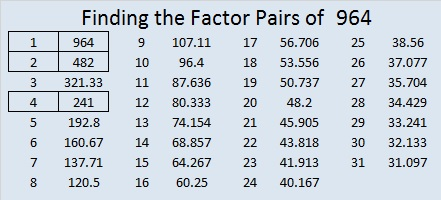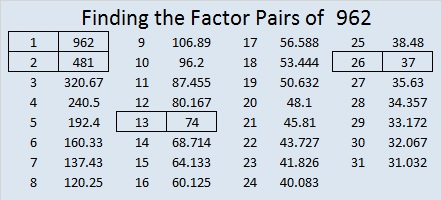964 Bow and Arrow

You can google lots of images of bows and arrows related to Thanksgiving. Perhaps that is because Indians from the Wampanoag Nation joined the Pilgrims in what we call the first Thanksgiving. I’m sure you know the story very well told from the white man’s point of view. It will be well worth your time to read The REAL Story of Thanksgiving as well. Everyone’s History Matters is also an excellent read.Print the puzzles or type the solution in this excel file: 12 factors 959-967

Now I’ll tell you a little about the number 964:

964 is the sum of the fourteen prime numbers from 41 to 101.

It is also the sum of four consecutive prime numbers:
233 + 239 + 241 + 251 = 964

30² + 8²  = 964 making it the hypotenuse of a Pythagorean triple:
480-836-964 which is 4 times (120-209-241)

964 is a palindrome in 2 bases and a repdigit in another.
1022201 in BASE 3
7A7 in BASE 11 (A is 10 in base 10)
444 in BASE 15

• 964 is a composite number.
• Prime factorization: 964 = 2 × 2 × 241, which can be written 964 = 2² × 241
• The exponents in the prime factorization are 2 and 1. Adding one to each and multiplying we get (2 + 1)(1 + 1) = 3 × 2  = 6. Therefore 964 has exactly 6 factors.
• Factors of 964: 1, 2, 4, 241, 482, 964
• Factor pairs: 964 = 1 × 964, 2 × 482, or 4 × 241
• Taking the factor pair with the largest square number factor, we get √964 = (√4)(√241) = 2√241 ≈ 31.048349962 Buckle Up, Pilgrim

Perhaps the most distinctive element of the pilgrims’ wardrobe is the buckle. We see it around the men’s waists, on their shoes, and on their hats. At least we do we if are looking at pilgrim costumes. It probably didn’t play as prominent a role on their actual clothes. Nevertheless, it is featured here on today’s puzzle. So buckle up and see where this puzzle takes you.Print the puzzles or type the solution in this excel file: 12 factors 959-967

Here are a few facts about the number 962:

It is the sum of two squares two different ways:
31² +  1² = 962
29² +  11² =  962

It is also the hypotenuse of four Pythagorean triples:
62-960-962, calculated from 2(31)(1), 31² –  1², 31² +  1²
312-910-962, which is 2 times (156-455-481)
370-888-962, which is 2 times (185-444-481)
638-720-962, calculated from 2(29)(11), 29² –  11², 29² +  11²

It looks interesting in a few other bases:
4242 BASE 6
282 BASE 20
101 BASE 31
QQ BASE 36 (Q is 26 in base 10)

• 962 is a composite number.
• Prime factorization: 962 = 2 × 13 × 37
• The exponents in the prime factorization are 1, 1, and 1. Adding one to each and multiplying we get (1 + 1)(1 + 1)(1 + 1) = 2 × 2 × 2 = 8. Therefore 962 has exactly 8 factors.
• Factors of 962: 1, 2, 13, 26, 37, 74, 481, 962
• Factor pairs: 962 = 1 × 962, 2 × 481, 13 × 74, or 26 × 37
• 962 has no square factors that allow its square root to be simplified. √962 ≈ 31.01612